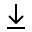MathBin Hyperbola Member

An hyperbola is a set of points such that the absolute difference to two fixed points (the foci) is constant, usually denoted by 2a.

Here are the common conventions for the hyperbola bins.

• The ContourPlot method plots the hyperbola in blue.
• The center is the purple point.
• The two vertices are the blue points.
• The two foci are the black points.
• The two directrices are the dark green dashed lines.
• The two latus rectums are the brown dashed line segments.
• The two end points of the conjugate axis are the dark gray points.
• The two asymptotes of the hyperbola are the gray dashed lines.
• You can manually zoom out of the graph.
Bins in Two Dimensions